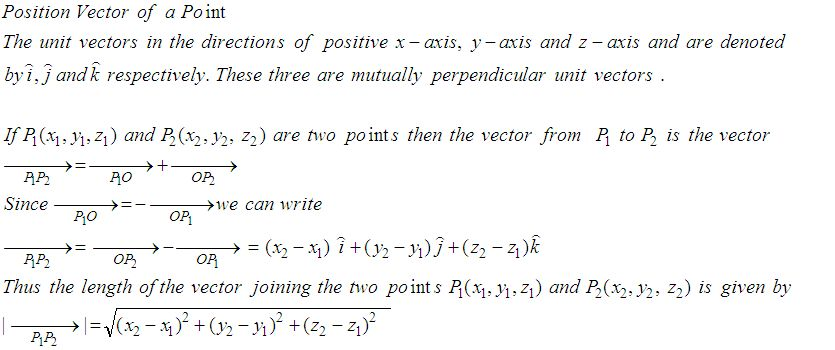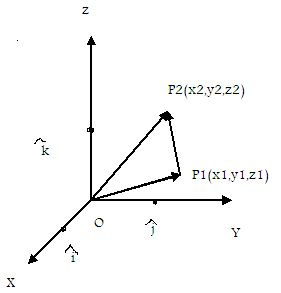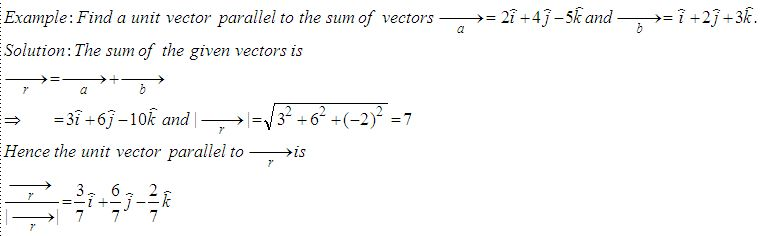Name: ___________________Date:___________________

 Email us to get an instant 20% discount on highly effective K-12 Math & English kwizNET Programs!

High School Mathematics - 25.9 Position Vector of a PointDirections: Answer the following.
 Q 1: Find the unit vector in the direction of of P(1,2) and Q(4,5).2i+5j(i+j)/Ö23i-2j Q 2: If P = 2,4,7 and P2 = (-4, -1, 5) then find the magnitude of vector P1P2.6i+5jÖ653i+6j Q 3: Find a unit vector in the direction from P(3,2) towards Q(5,6).i/Ö5 + 2j/Ö54i+52i+3 Q 4: Find the vector with initial point P(6,-2) and terminal point Q(4,-8).6i-9j-2i+10j8i+2j Q 5: If P = 2,4,7 and P2 = (-4, -1, 5) then find vector P1P2.-6i-5j-2k-6i+5j6i+5j+3k Q 6: Find the unit vector in the direction of P(1,2,3) towards Q(4,5,6).8j-2k(i+j+k)/Ö36i+5j+2k Q 7: Find a unit vector parallel to the sum of vectors a = 2i+4j-5k, b = i+2j+3k3/7i + 6/7j - 2/7 k8i+4j-3k2-i8 Q 8: Find the condition that the vectors a = ki + 3j and b = 4i+kj, (k not equal to 0) are parallel.k2 = 12k = 6k = 0 Question 9: This question is available to subscribers only! Question 10: This question is available to subscribers only!